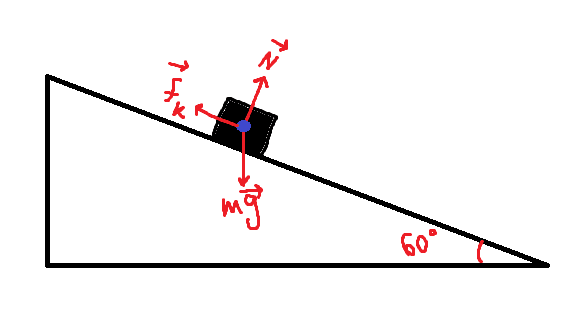# How to find coefficient of friction of a body sliding down the slope?

• Callmelucky
In summary, the conversation discusses finding the coefficient of friction using two different approaches and obtaining different results. The confusion arises from the assumption that the coefficient of friction cannot be greater than 1 when the body is moving. However, it is clarified that this is not always the case. The conversation also mentions using a coordinate system and applying the net force equation to find the necessary equations. It is also mentioned that the textbook used is a workbook by Nada Brkovic, which has a question about the coefficient of friction being greater than 1, but it is not properly answered. f

#### Callmelucky

Homework Statement
Body starts to slide down the 60degree incline plane and after 20 meters reaches speed of 4 m/s. What is the coefficient of friction?
Relevant Equations
acceleration due to gravity under angle ->Fg=mgsin60, Normal force -> Fn=mgcos60, Ffriction = mi(coefficient of friction)*Fn
So basically I need to find the coefficient of friction given the listed information.
What bothers me is that I am getting two different accelerations for two different approaches. When I calculate acceleration using Fg=mgsin60 I do it this way: Fg=mgsin60 -> ma=mgsin60 ->a=gsin60 -> a=8.66. But when I use formula ##v^2=2as## I get a=0.4.

After I got a using Fg=mgsin60 I use Ftr=Fn*mi -> ma=mgcos60*mi -> ##\frac{a}{gcos60}=mi## -> mi(fr coef)= 1.73. Which makes no sense, since body is moving and coef of friction can't be greater or equal to 1 if body is moving.

And using a I got from ##v^2=2as## -> ##\frac{a}{gcos60}=mi## -> mi= 0.08 and that makes sense, BUT the solution in the textbook is 1.65, so I am really confused. I suppose it's mistake in textbook, but why am I getting two different soltions for a?

So yeah, if you could please explain I would be very grateful,
thank you.

Fg=mgsin60 -> ma=mgsin60
That assumes no friction. Try including the frictional force in the equation.

•MatinSAR, Callmelucky and erobz
. . . and coef of friction can't be greater or equal to 1 if body is moving.
Why?

•Callmelucky and MatinSAR

### how to find coefficient of friction of a body sliding down the slop?​

Just FYI, most slop has a VERY low coefficient of friction.

•kuruman
You want to find coefficient of friction. But you have forgotten the firiction force in your answer!Try to add a X-Y coordinate system and find component of each force in ##x## and ##y## direction. Then apply ##\vec F_{net}=m\vec a## ! You should know that the mentioned vector equation equivalent to three component equations! So you can find equations you have mentioned in post #1 without forgetting the firiction force.

•Callmelucky
@MatinSAR, @haruspex. I got it, thank you.
@kuruman, I had no idea that mi can be >=1, there was a question in the textbook if mi can be >= 1 and the answer at the end was that it can not. I never checked that, just accepted it as a fact, but after checking I realized that it obviously can be, thank you for telling me.

•MatinSAR and kuruman
@MatinSAR, @haruspex. I got it, thank you.
@kuruman, I had no idea that mi can be >=1, there was a question in the textbook if mi can be >= 1 and the answer at the end was that it can not. I never checked that, just accepted it as a fact, but after checking I realized that it obviously can be, thank you for telling me.
What text book?

What text book?
it's actually a workbook by Nada Brkovic, there is a question(273) if the friction coefficient can be greater than 1, and, somehow I concluded that it can not be greater than 1 if the body is moving. The best thing is that it is not a proper question, it's more like a side question along with 3 main questions and it is not actually answered at the end of the textbook. I don't know why I thought that it was answered and that the answer was that coef of friction can't be >=1.